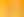The X Factor: Using Algebra to Solve Problems

# Stages1. Paths in the Park

EXPLORE

Suggested Learning Intentions

• To explore, construct and explain generalisations representing a relationship between two variables

Sample Success Criteria

• I can use manipulatives to model a pattern or relationship
• I can construct my own generalisation to represent a pattern or relationship
• I can explain my generalisation to another person
• I can write my generalisation using mathematical language
• I can use my generalisation to solve problems
• I can justify my solutions using a range of manipulatives
EXPLORE

2. Garden Beds

EXPLORE

Suggested Learning Intentions

• To explore, construct and explain generalisations representing relationships between two variables
• To understand and justify that there can be different ways of writing a generalisation

Sample Success Criteria

• I can model a problem using manipulatives and find a solution
• I can construct at least two generalisations to represent a pattern or relationship
• I can explain why these are valid in the context of the problem
• I can draw a graph of the relationship
• I can justify my solution using a variety of manipulatives
EXPLORE

3. Snail Trail

EXPLORE

Suggested Learning Intentions

• To complete a mathematical investigation involving variables
• To successfully apply a range of problem-solving strategies to a given task

Sample Success Criteria

• I can use a range of problem-solving strategies to help me solve a problem
• I can use materials to model a problem
• I can identify and justify which strategies are most useful
• I can complete a mathematical investigation involving variables
• I can justify my solution using a range of manipulatives
EXPLORE

4. Chocolate Boxes

EXPLORE

Suggested Learning Intentions

• To complete a mathematical investigation involving variables
• To successfully apply a range of problem-solving strategies to a given task

Sample Success Criteria

• I can use a range of problem-solving strategies to help me solve a problem
• I can model a problem using materials
• I can identify and justify which strategies are most useful
• I can justify my solution using a range of manipulatives
EXPLORE